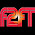## Tuesday, June 30, 2015

### 122 142 | Indonesia Plane Crash Reporting

122 people on board?  Talk about a hot number...
• Confederate Flag = 122
• Pope Francis = 122
• Golden State = 122
• San Francisco = 122
142 is also a number of significance.
• Terrorist = 142
• Forty Two = 142
The victims were treated at 'Adam Malik General Hospital'.
• Adam = 1+4+1+4 = 10
• Malik = 4+1+3+9+2 = 19
• General = 7+5+5+5+9+1+3 = 35
• Hospital = 8+6+1+7+9+2+1+3 = 37/46
• Adam Malik General Hospital = 101/110
Medan...
• Medan = 4+5+4+1+5 = 19
• Medan = 13+5+4+1+14 = 37
Major General Fuad Basya?
• Major = 4+1+1+6+9 = 21
• General = 7+5+5+5+9+1+3 = 35
• Fuad = 6+3+1+4 = 14
• Basya = 2+1+1+7+1 = 12/21
• Major General Fuad Basya = 82/91
September 11 happened on a Tuesday.

#### 2 comments:

1.This happened 98 days after the Germanwings crash...

1.LOL, I meant to look at that, glad you did!